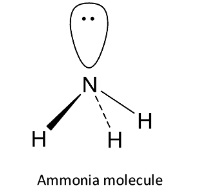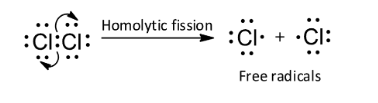QuestionAnswers

# Ammonia molecule is:(A) A nucleophile(B) An electron deficient molecule(C) A homolytic molecule(D) An acid

Hint: Ammonia is a volatile trihydride of the element nitrogen of group 15. It has the formula ${\text{N}}{{\text{H}}_{\text{3}}}$ . Nucleophiles or nucleophilic reagents are attracted to the nucleus or are nucleus loving or positive charge loving. Thus, the nucleophiles can be said to be the type of reagent species which are rich in electrons. Thus, the nucleophiles are negatively charged species.
Nucleophiles are of two types, negative nucleophiles or neutral nucleophiles. According to the Lewis concept, a nucleophile can donate an electron pair and hence it acts as a Lewis base.

The neutral nucleophiles are those nucleophiles which have an unshared pair of electrons but do not carry any charge. Now, let us see the structure of the ammonia molecule. The valence shell configuration of nitrogen is ${\text{2}}{{\text{s}}^{\text{2}}}{\text{2}}{{\text{p}}^{\text{3}}}$ and it undergoes ${\text{s}}{{\text{p}}^{\text{3}}}$ hybridization. Three of the four ${\text{s}}{{\text{p}}^{\text{3}}}$ hybrid orbitals overlap with 1s orbitals of 3 hydrogen atoms to form 3 ${\text{N - H}}$ sigma bonds while the fourth contains the lone pair of electrons. It has pyramidal structure.